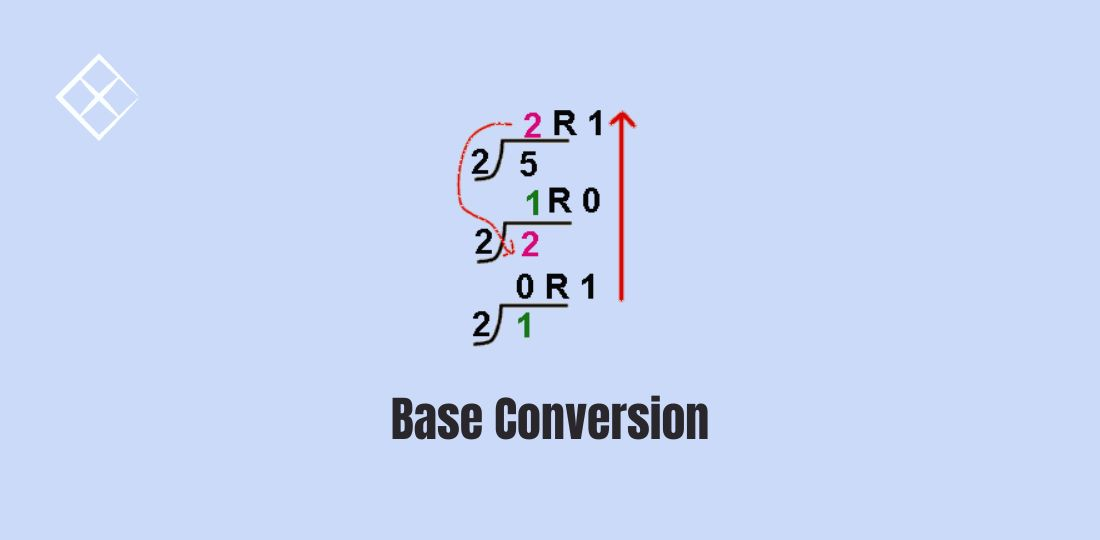# Base Conversion## Problem

Given an integer `num`, return a string of its base 7 representation.

``````Example 1:
Input: num = 100
Output: "202"

Example 2:
Input: num = -7
Output: "-10"
``````

### Positive Case

Base conversion can be done through a succession of Euclidean Division. We repeatedly divide `n` by base and store the remainder. Finally we reverse the obtained string.

``````def to_base_7_positive(num: int) -> str:
"""assume num is positive"""
result = []
while num:
num, r = divmod(num, 7)
result.append(str(r))
result.reverse()
return "".join(result)
``````

### General Case

Of course, when `n` is 0, the output should also be 0. When `n` is negative, the result of the conversion should be the negation of the positive case:

``````def convertToBase7(num: int) -> str:
if num == 0:
return "0"
if num > 0:
else:
return "-" + to_base_7_positive(-num)
``````

## Another Example

Given an integer num, return a string representing its hexadecimal representation.

For negative integers, two’s complement method is used.

All the letters in the answer string should be lowercase characters, and there should not be any leading zeros in the answer except for the zero itself.

``````Example 1:
Input: num = 26
Output: "1a"

Example 2:
Input: num = -1
Output: "ffffffff"
``````

#### Solution

``````def to_hex(num: int) -> str:
if num == 0:
return "0"
if num > 0:
else:

def to_hex_positive(num):
transform = '0123456789abcdef'
res = []
while num:
num, r = divmod(num, 16)
res.append(transform[r])
res.reverse()
return "".join(res)
``````

### The Reverse

How can we do the reverse? Given a number and its base, how do we convert it to decimal?

Let’s do some examples. `1234` in base 5 equals `1*5^3+2*5^2+3*5+4=194` in base 10. `6a9` in hex equals `6*16^2+10*16+9=1705` in decimal.

#### Excel Sheet Column Number

``````Given a string columnTitle that represents the column title as appear in an Excel sheet, return its corresponding column number.

For example:

A -> 1
B -> 2
C -> 3
...
Z -> 26
AA -> 27
AB -> 28
...

Example 1:
Input: columnTitle = "A"
Output: 1

Example 2:
Input: columnTitle = "AB"
Output: 28

Example 3:
Input: columnTitle = "ZY"
Output: 701
``````

#### Solution

``````def titleToNumber(s: str) -> int:
return sum(
(ord(char) - ord('A') + 1) * 26 ** i
for i, char in enumerate(reversed(s))
)
``````